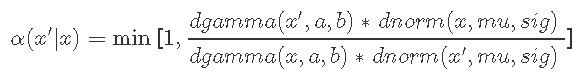# R语言MCMC:Metropolis-Hastings采样用于回归的贝叶斯估计

## MCMC是从复杂概率模型中采样的通用技术。

1.蒙特卡洛

2.马尔可夫链

3.Metropolis-Hastings算法

1.1直接模拟

1.2逆CDF

1.3拒绝/接受抽样

### 马尔可夫链

马尔可夫链的收敛性意味着它具有平稳分布π。马尔可夫链的统计分布是平稳的,那么它意味着分布不会随着时间的推移而改变。

# Metropolis算法

对于一个Markov链是平稳。基本上表示

# Metropolis-Hastings算法

A的选择遵循以下逻辑。

1. 初始化：随机选择一个初始状态x；
2. 根据q（x’| x）随机选择一个新状态x’;

3.接受根据α（x’| x）的状态。如果不接受，则不会进行转移，因此无需更新任何内容。否则，转移为x’；

4.转移到2，直到生成T状态；

5.保存状态x，执行2。

### 属性

Metropolis-Hastings算法的一个有趣特性是它 仅取决于比率

# Metropolis采样

### 一个简单的Metropolis-Hastings采样

1. 从某种状态开始xt。代码中的x。
2. 在代码中提出一个新的状态x’候选
3. 计算“接受概率”4. 从[0,1] 得出一些均匀分布的随机数u；如果u <α接受该点，则设置xt + 1 = x’。否则，拒绝它并设置xt + 1 = xt。

# MH可视化

set.seed(123)

for (i in 2:n) {
can <- rnorm(1, mu, sig)
aprob <- min(1, (dgamma(can, a, b)/dgamma(x,
a, b))/(dnorm(can, mu, sig)/dnorm(x,
mu, sig)))
u <- runif(1)
if (u < aprob)
x <- can
vec[i] <- x

### 画图

nrep<- 54000
burnin<- 4000
shape<- 2.5
rate<-2.6

vec=vec[-(1:burnin)]
#vec=vec[burnin:length(vec)]
par(mfrow=c(2,1)) # 更改主框架，在一帧中有多少个图形
plot(ts(vec), xlab="Chain", ylab="Draws")
abline(h = mean(vec), lwd="2", col="red" )
    Min.  1st Qu.   Median     Mean  3rd Qu.     Max.
0.007013 0.435600 0.724800 0.843300 1.133000 3.149000 
var(vec[-(1:burnin)])
 0.2976507

# 第一个样本 vec 是我们链的初始/起始值。我们可以更改它，以查看收敛是否发生了变化。

        x <- 3*a/b
vec <- x

# 示例2：回归的贝叶斯估计

Metropolis-Hastings采样用于贝叶斯估计回归模型。

### DGP和图

# 创建独立的x值，大约为零
x <- (-(Size-1)/2):((Size-1)/2)
# 根据ax + b + N（0，sd）创建相关值
y <-  trueA * x + trueB + rnorm(n=Size,mean=0,sd=trueSd)

### 正态分布拟然


pred = a*x + b
singlelikelihoods = dnorm(y, mean = pred, sd = sd, log = T)
sumll = sum(singlelikelihoods)

# 示例：绘制斜率a的似然曲线

# 示例：绘制斜率a的似然曲线
plot (seq(3, 7, by=.05), slopelikelihoods , type="l")

### 先验分布

# 先验分布

# 更改优先级，log为True，因此这些均为log
density/likelihood
aprior = dunif(a, min=0, max=10, log = T)
bprior = dnorm(b, sd = 2, log = T)
sdprior = dunif(sd, min=0, max=30, log = T)

### 后验

posterior <- function(param){
return (likelihood(param) + prior(param))
}

# Metropolis算法

1. 从随机参数值开始
2. 根据某个候选函数的概率密度，选择一个接近旧值的新参数值
3. 以概率p（new）/ p（old）跳到这个新点，其中p是目标函数，并且p> 1也意味着跳跃
4. 请注意，我们有一个 对称的跳跃/候选分布 q（x’| x）。

######## Metropolis 算法 ################

for (i in 1:iterations){

probab = exp(posterior(proposal) - posterior(chain[i,]))
if (runif(1) < probab){
chain[i+1,] = proposal
}else{
chain[i+1,] = chain[i,]
}

### 实施

（e）输出接受的值，并解释。


chain = metrMCMC(startvalue, 5500)

burnIn = 5000
accep = 1-mean(duplicated(chain[-(1:burnIn),]))

startvalue = c(4,0,10)

### 小结

       V1              V2                V3
Min.   :4.068   Min.   :-6.7072   Min.   : 6.787
1st Qu.:4.913   1st Qu.:-2.6973   1st Qu.: 9.323
Median :5.052   Median :-1.7551   Median :10.178
Mean   :5.052   Mean   :-1.7377   Mean   :10.385
3rd Qu.:5.193   3rd Qu.:-0.8134   3rd Qu.:11.166
Max.   :5.989   Max.   : 4.8425   Max.   :19.223  
#比较:
summary(lm(y~x))

Call:
lm(formula = y ~ x)

Residuals:
Min      1Q  Median      3Q     Max
-22.259  -6.032  -1.718   6.955  19.892

Coefficients:
Estimate Std. Error t value Pr(>|t|)
(Intercept)  -3.1756     1.7566  -1.808    0.081 .
x             5.0469     0.1964  25.697   <2e-16 ***
---
Signif. codes:  0 ?**?0.001 ?*?0.01 ??0.05 ??0.1 ??1

Residual standard error: 9.78 on 29 degrees of freedom
Multiple R-squared:  0.9579,    Adjusted R-squared:  0.9565
F-statistic: 660.4 on 1 and 29 DF,  p-value: < 2.2e-16
summary(lm(y~x))\$sigma
 9.780494
coefficients(lm(y~x))
(Intercept)
-3.175555 
coefficients(lm(y~x))
       x
5.046873 

### 总结：

### 总结: #######################

par(mfrow = c(2,3))
hist(chain[-(1:burnIn),1],prob=TRUE,nclass=30,col="109"
abline(v = mean(chain[-(1:burnIn),1]), lwd="2")

Kaizong Ye拓端研究室（TRL）的研究员。

​非常感谢您阅读本文，如需帮助请联系我们！QQ在线咨询

15121130882

0571-63341498

## 关注有关新文章的微信公众号

This will close in 0 seconds